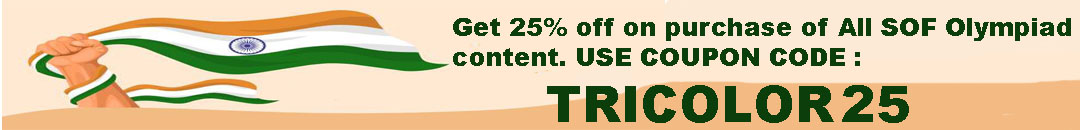# User ForumSubject :IMO    Class : Class 3

## Ans 1:

Class : Class 7

Subject :IMO    Class : Class 6

Subject :IMO    Class : Class 6

## Ans 1:

Class : Class 6
Let each have Rs. x .After buying a dress Priya has Rs.(x â 79.90)After buying a watch Amit has Rs.(x â 193.40)Given, (x â 79.90) = 3( x â 193.40)So, x â 79.90 = 3x - 580.203x -580.20 = x â 79.903x â x = Rs. 580.20 - 79.902x = Rs. 500.30So, x = Rs. 500.30/2 = Rs. 250.15Answer-(D) Rs. 250.15

Subject :IMO    Class : Class 6

Subject :IMO    Class : Class 6

Subject :IMO    Class : Class 6

Subject :IMO    Class : Class 6

Subject :IMO    Class : Class 9

## Ans 1:

Class : Class 9

Subject :IMO    Class : Class 4

Class : Class 4

## Ans 2:

Class : Class 7
answer is a we know that 1 football= 5 tennis balls and a gift box 2 gift box= 10 tennis balls so we can say that football is f and tennis ball is t and gift box is g 2g=10t so 1g=5t so 1f =5t and 1g or 1f=5t+5t=10t or 2g so 1f =10t 1 football equals to 10 tennis balls